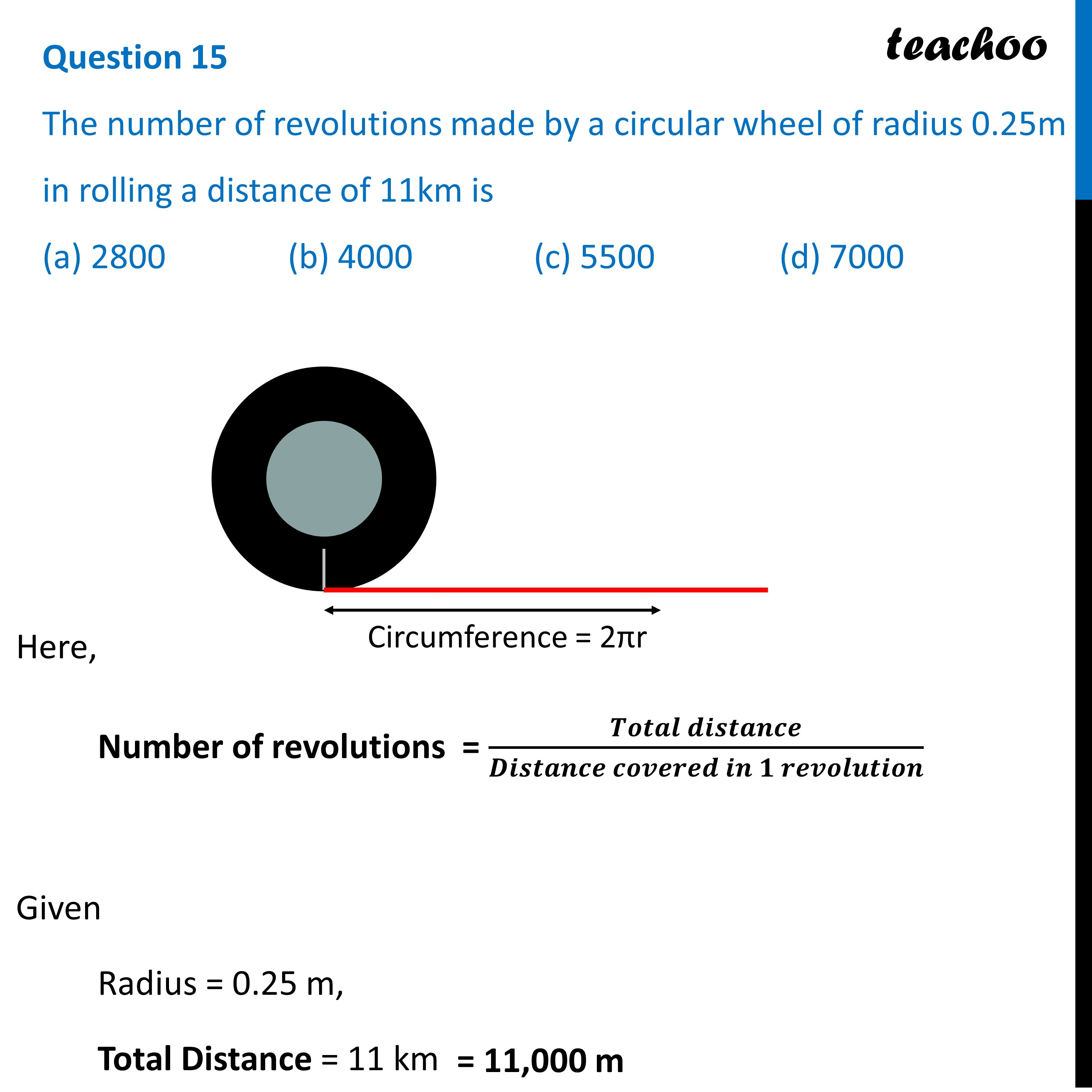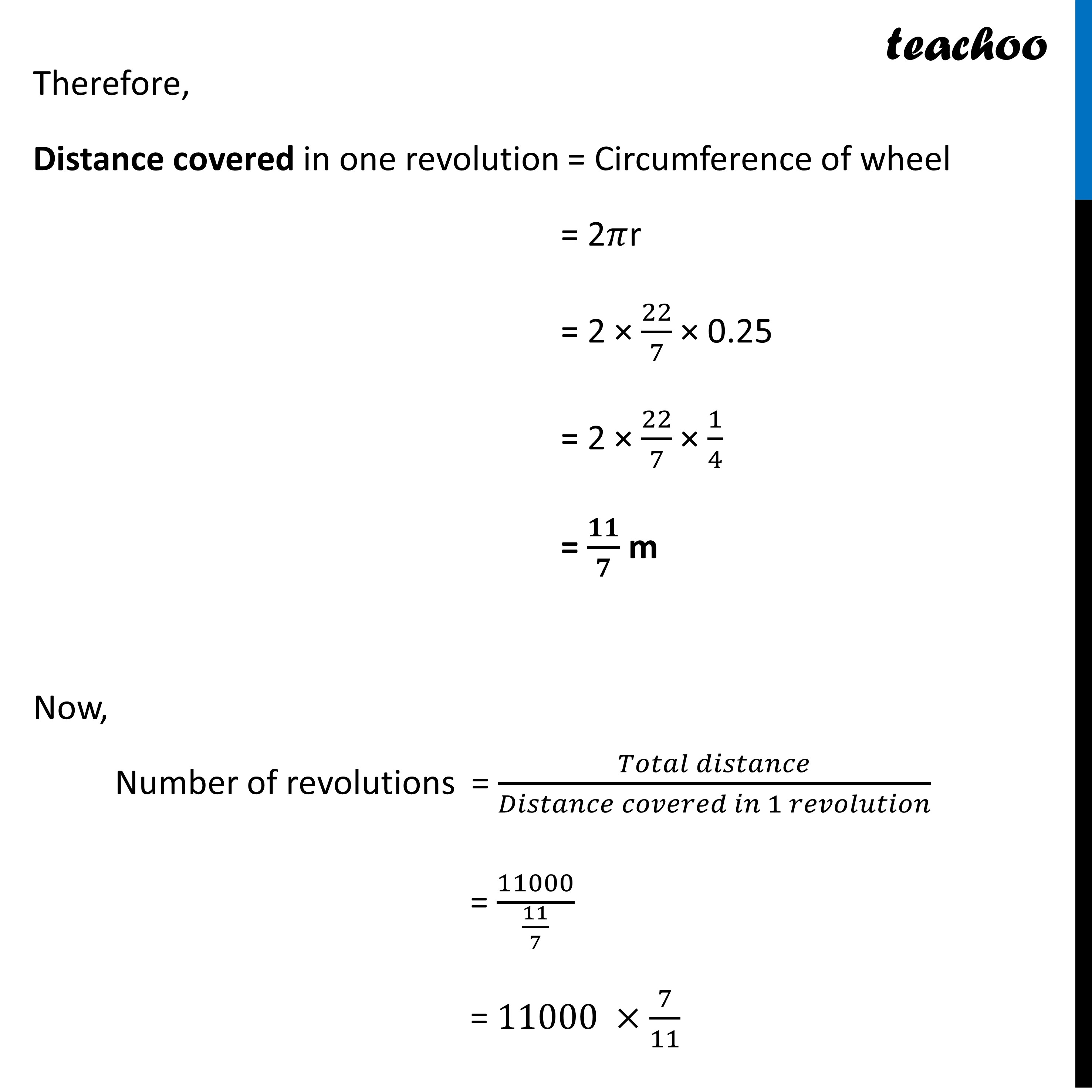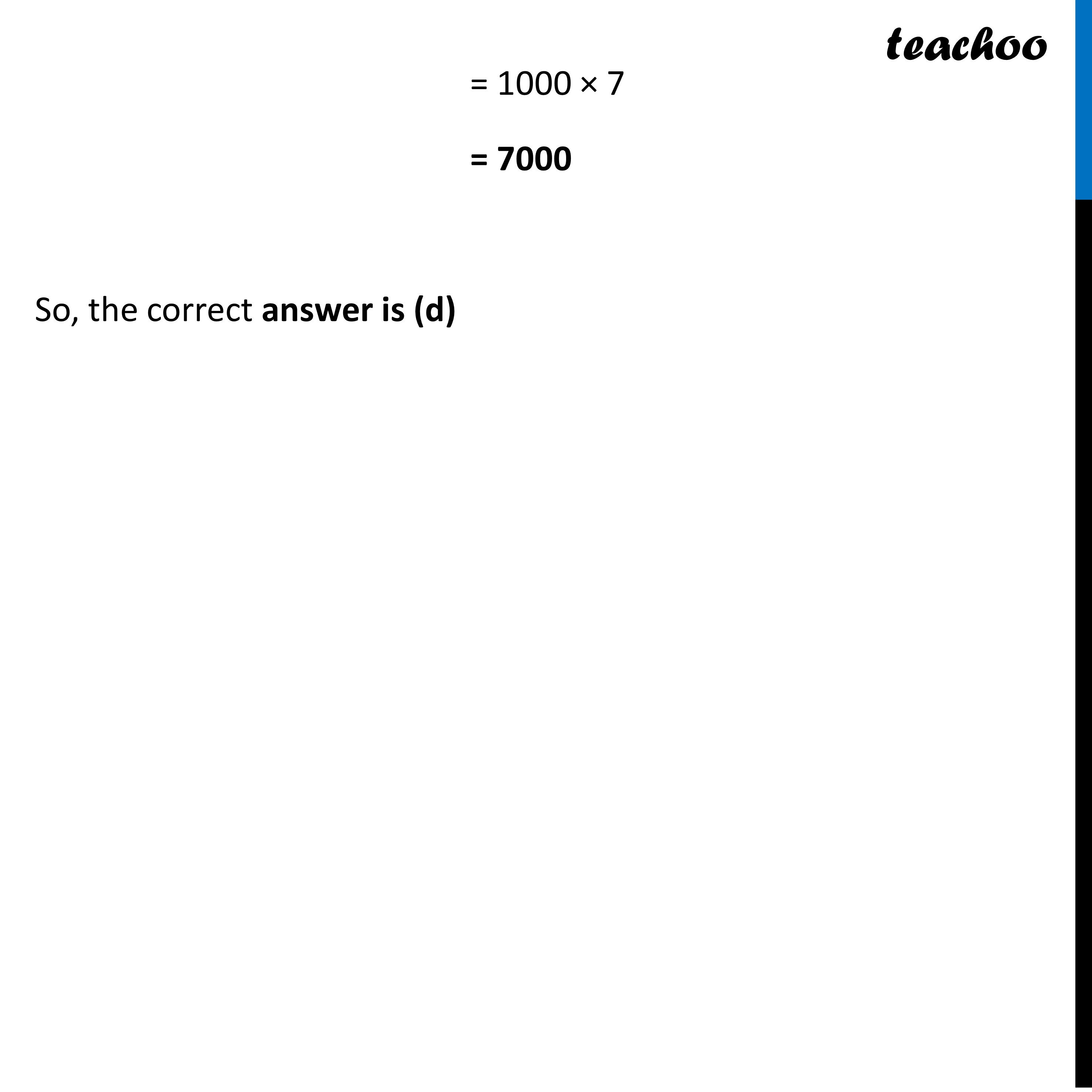CBSE Class 10 Sample Paper for 2023 Boards - Maths Standard

Class 10
Solutions of Sample Papers for Class 10 Boards

## (a) 2800   (b) 4000   (c) 5500   (d) 7000Learn in your speed, with individual attention - Teachoo Maths 1-on-1 Class

### Transcript

Question 15 The number of revolutions made by a circular wheel of radius 0.25m in rolling a distance of 11km is (a) 2800 (b) 4000 (c) 5500 (d) 7000 Here, Number of revolutions = (𝑻𝒐𝒕𝒂𝒍 𝒅𝒊𝒔𝒕𝒂𝒏𝒄𝒆)/(𝑫𝒊𝒔𝒕𝒂𝒏𝒄𝒆 𝒄𝒐𝒗𝒆𝒓𝒆𝒅 𝒊𝒏 𝟏 𝒓𝒆𝒗𝒐𝒍𝒖𝒕𝒊𝒐𝒏) Given Radius = 0.25 m, Total Distance = 11 km Therefore, Distance covered in one revolution = Circumference of wheel = 2𝜋r = 2 × 22/7 × 0.25 = 2 × 22/7 × 1/4 = 𝟏𝟏/𝟕 m Now, Number of revolutions = (𝑇𝑜𝑡𝑎𝑙 𝑑𝑖𝑠𝑡𝑎𝑛𝑐𝑒)/(𝐷𝑖𝑠𝑡𝑎𝑛𝑐𝑒 𝑐𝑜𝑣𝑒𝑟𝑒𝑑 𝑖𝑛 1 𝑟𝑒𝑣𝑜𝑙𝑢𝑡𝑖𝑜𝑛) = 11000/(11/7) = 11000 ×7/11 = 1000 × 7 = 7000 So, the correct answer is (d)